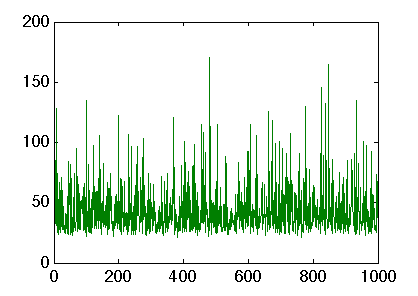# Stable Matchings

### Contents

Related publications: [BN12]

### Introduction

The Stable Roommates problem is a classical combinatorial problem (SR) that has been studied extensively. The SR problem and its variants arise in many practical applications, such as matching graduating medical students to hospital posts, residents or in college admissions.

An instance I of SR contains an undirected graph G(V, E), where V = {v1, . . . , vn} and m=|E(G)|. We refer to G as the underlying graph of I, and the vertices of G as the agents. If (u, v) is an edge in G(V, E), then we say that u and v find each other acceptable. Each agent u has a linear order >u over her acceptable partners, where v >u w means that u prefers v to w. Let M(u) denote the partner of u in a given matching M. An edge (u,v) is said to be blocking with respect to M if:

• either u is unmatched in M or prefers v to M(u);

• either v is unmatched in M or prefers u to M(v).

A matching is called stable if it admits no blocking edge.

If G is bipartite, then the problem of finding a stable matching is called the Stable Marriage problem (SM) In this case, if the graph is G(U,V,E), then we refer to U={m1, . . . , mn1} and W ={w1, . . . , wn2 } as the sets of men and women, respectively.

Knuth [Knu76] asked the following question (Problem 8 from his twelve famous research problems). Suppose that we are given an instance of SM with complete lists and an unstable matching M . If (m,w) is a blocking pair for M , then a matching can be obtained from M and (m,w) by an interchange, i.e., by matching the blocking pair and matching their partners to each other. Does there always exist a sequence of interchanges from M to some stable matching?

Although Tamura [Tam93] answers Knuth's question negatively by giving a counterexample, Roth and Vande Vate [RV90] show that, given an instance of SR, starting from any unstable matching we always obtain a stable matching by successively satisfying blocking pairs, i.e., by adding the blocking pair to the matching and leaving their partners (if there were any) unmatched. Diamantoudi et al. [DMX04] show a similar result holds for the roommates problem, namely, for a given instance of sr that admits a stable matching and starting from any unstable matching, one obtains a stable matching by successively satisfying blocking pairs.

We study the dynamics of matching markets where two agents meet with each other randomly and behave in a myopic way (they form a new pair if they both would be better off). More precisely, we suppose that in each step of the process, each blocking edge is chosen with equal probability and then the matching is updated to satisfy the blocking pair. This is called the better response dynamics by Ackermann et al. [AGM+08], while Klaus et al. [KKW08] refer to it as unperturbed blocking dynamics.

### Example 1 (Gale & Shapley)

We start with a classical instance by Gale and Shapley [GS62] with three men and three women and the following preferences:

• m1 : w1,w2,w3
• m2 : w2,w3,w1
• m1 : w1,w2,w3
• w1 : m2,m3,m1
• w2 : m3,m1,m2
• w3 : m1,m2,m3

The PRISM source code for this instance is given below.

```// stable marriage example [GS62]
// gxn 15/04/10

// we construct the model as a set of modules run in parallel, where each module corresponds to
// one man or one woman and where the actions correspond to satisfying blocking pairs

// for example action eij corresponds to the current matching being updated due to man i
// and woman j being a blocking pair, therefore the action should only be possible if both
// man i and woman j satisfy the conditions of a blocking pair
// this is indeed the case as the guards in the modules of man i and woman j for action eij
// when combined match the condition of a blocking pair

// since satisfying a blocking pair can change the matching of other agents, we include these
// actions in the other agents so they can update there matching accordingly
// for example if eij is performed and woman k was mached with man i, then after
// satisfying the blocking pair woman k will not be matched
// on the other hand if woman k was not matched with man i, then nothing would change

// since PRISM automatically normalises the transition probabilities when constructing DTMCs,
// by giving each transition probability 1 (the default value so no probability needs to be
// verified), in the constructed model there will be a uniform probabilistic over the enabled transitions
// i.e. a uniform probabilistic choice between the current block pairs which corresponds precisely
// with the "better response dynamics" of Ackermann et al. [AGM+08] and the "unperturbed blocking dynamics"
// of Klaus et al. [KKW08]

// note we do not add loops in stable matchings, as using the fact that the stable matchings
// are deadlocks states (states with no outgoing transitions) we can use the label "deadlock"
// to specify the stable matchings, and hence simplify the specification of properties

dtmc // model is a DTMC/Markov chain

//------------------------------------------------------
// PREFERENCE LISTS
// man i prefers woman j over woman k if mij>mik
// woman i prefers man j over man k if wij>wik

// preference list for men
const int m11=3;
const int m12=2;
const int m13=1;

const int m22=3;
const int m23=2;
const int m21=1;

const int m33=3;
const int m31=2;
const int m32=1;

// preference list for women
const int w12=3;
const int w13=2;
const int w11=1;

const int w23=3;
const int w21=2;
const int w22=1;

const int w31=3;
const int w32=2;
const int w33=1;

//------------------------------------------------------
// constants used in renaming
const int N1=1;
const int N2=2;
const int N3=3;

//------------------------------------------------------
// module for first man
module man1

// current matching (0 means no matching)
m1 : [0..3];

// wants to change matching
[e11] m1=0 | (m1=1 & m11>m11)|(m1=2 & m11>m12)|(m1=3 & m11>m13) -> (m1'=1);
[e12] m1=0 | (m1=1 & m12>m11)|(m1=2 & m12>m12)|(m1=3 & m12>m13) -> (m1'=2);
[e13] m1=0 | (m1=1 & m13>m11)|(m1=2 & m13>m12)|(m1=3 & m13>m13) -> (m1'=3);

// one of the other pairs change so may need to reset matching
[e21] true -> (m1'=(m1=1)?0:m1);
[e31] true -> (m1'=(m1=1)?0:m1);
[e22] true -> (m1'=(m1=2)?0:m1);
[e32] true -> (m1'=(m1=2)?0:m1);
[e23] true -> (m1'=(m1=3)?0:m1);
[e33] true -> (m1'=(m1=3)?0:m1);

endmodule

// construct further men through renaming
module man2=man1[m1=m2, m11=m21, e11=e21, e12=e22, e13=e23, m12=m22, e21=e31, e22=e32, e23=e33, m13=m23, e31=e11, e32=e12, e33=e13 ] endmodule
module man3=man1[m1=m3, m11=m31, e11=e31, e12=e32, e13=e33, m12=m32, e21=e11, e22=e12, e23=e13, m13=m33, e31=e21, e32=e22, e33=e23 ] endmodule

//------------------------------------------------------
// module for first woman
module woman1

// do not need to store the matching (can work it out from the men's variables)

// man 1 wants to change
[e11] ( m1!=N1 & m2!=N1 & m3!=N1 ) | (m1=N1 & w11>w11)|(m2=N1 & w11>w12)|(m3=N1 & w11>w13) -> true;
[e21] ( m1!=N1 & m2!=N1 & m3!=N1 ) | (m1=N1 & w12>w11)|(m2=N1 & w12>w12)|(m3=N1 & w12>w13) -> true;
[e31] ( m1!=N1 & m2!=N1 & m3!=N1 ) | (m1=N1 & w13>w11)|(m2=N1 & w13>w12)|(m3=N1 & w13>w13) -> true;

endmodule

// construct further women through renaming
module woman2=woman1[ N1=N2, w11=w21, e11=e12, e21=e22, e31=e32, w12=w22, e12=e13, e22=e23, e32=e33, w13=w23, e13=e11, e23=e21, e33=e31 ] endmodule
module woman3=woman1[ N1=N3, w11=w31, e11=e13, e21=e23, e31=e33, w12=w32, e12=e11, e22=e21, e32=e31, w13=w33, e13=e12, e23=e22, e33=e32 ] endmodule

//------------------------------------------------------
// reward structure: expected rounds
rewards "rounds"

true : 1;
endrewards
```

Constructing the model in PRISM we find the Markov chain has 34 states, 123 transitions, and the following deadlock/absorbing states:

• 17:(1,2,3)
• 26:(2,3,1)
• 31:(3,1,2)

which correspond to the stable matchings:

• Mm = {(m1, w1), (m2,w2), (m3,w3)} (man-optimal);
• Me ={(m1, w2), (m2,w3), (m3, w1)} (egalitarian);
• Mw = {(m1, w3), (m2,w1), (m3,w2)} (woman-optimal).

Calculating the steady state probabilities, PRISM returns:

• 17:(1,2,3)=0.219529
• 26:(2,3,1)=0.560937
• 31:(3,1,2)=0.219529

which shows the egalitarian stable matching is therefore more likely than both the extreme solutions together.

Next, we study the convergence of this instance by verifying the following properties:

• the probability of reaching a stable matching within r rounds;

• the expected number of rounds to reach a stable matching.

These properties can be expressed in PRISM as follows.

```// probability reached a stable matching by round R
const int r; // bound of number of rounds

// expected number of rounds to reach a stable matching
```

Analysing these properties in PRISM we find the expected number of rounds equals 9.115955 and the probabilities are plotted in the graph below.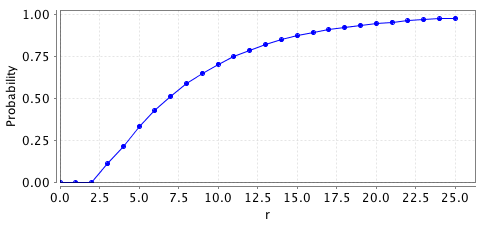### Example 2 (Knuth)

The following classical instance was proposed by Knuth [Knu76] with four men and four women and the following preferences:

• m1 : w1, w2, w3, w4
• m2 : w2, w1, w4, w3
• m3 : w3, w4, w1, w2
• m4 : w4, w3, w2, w1
• w1 : m4, m3, m2, m1
• w2 : m3, m4, m1, m2
• w3 : m2, m1, m4, m3
• w4 : m1, m2, m3, m4

The PRISM source code for this instance is given below.

```// stable marriage instance [Knu76]
// gxn 15/04/10

// we construct the model as a set of modules run in parallel, where each module corresponds to
// one man or one woman and where the actions correspond to satisfying blocking pairs

// for example action eij corresponds to the current matching being updated due to man i
// and woman j being a blocking pair, therefore the action should only be possible if both
// man i and woman j satisfy the conditions of a blocking pair
// this is indeed the case as the guards in the modules of man i and woman j for action eij
// when combined match the condition of a blocking pair

// since satisfying a blocking pair can change the matching of other agents, we include these
// actions in the other agents so they can update there matching accordingly
// for example if eij is performed and woman k was mached with man i, then after
// satisfying the blocking pair woman k will not be matched
// on the other hand if woman k was not matched with man i, then nothing would change

// since PRISM automatically normalises the transition probabilities when constructing DTMCs,
// by giving each transition probability 1 (the default value so no probability needs to be
// verified), in the constructed model there will be a uniform probabilistic over the enabled transitions
// i.e. a uniform probabilistic choice between the current block pairs which corresponds precisely
// with the "better response dynamics" of Ackermann et al. [AGM+08] and the "unperturbed blocking dynamics"
// of Klaus et al. [KKW08]

// note we do not add loops in stable matchings, as using the fact that the stable matchings
// are deadlocks states (states with no outgoing transitions) we can use the label "deadlock"
// to specify the stable matchings, and hence simplify the specification of properties

dtmc // model is a DTMC/Markov chain

//------------------------------------------------------
// PREFERENCE LISTS
// man i prefers woman j over woman k if mij>mik
// woman i prefers man j over man k if wij>wik

// preference list for men
const int m14=1;
const int m13=2;
const int m12=3;
const int m11=4;

const int m23=1;
const int m24=2;
const int m21=3;
const int m22=4;

const int m32=1;
const int m31=2;
const int m34=3;
const int m33=4;

const int m41=1;
const int m42=2;
const int m43=3;
const int m44=4;

// preference list for women
const int w11=1;
const int w12=2;
const int w13=3;
const int w14=4;

const int w22=1;
const int w21=2;
const int w24=3;
const int w23=4;

const int w33=1;
const int w34=2;
const int w31=3;
const int w32=4;

const int w44=1;
const int w43=2;
const int w42=3;
const int w41=4;

//------------------------------------------------------
// constants used in renaming
const int N1=1;
const int N2=2;
const int N3=3;
const int N4=4;

//------------------------------------------------------
// module for first man
module man1

// current matching (0 means no matching)
m1 : [0..4];

// wants to change matching
[e11] m1=0 | (m1=1 & m11>m11)|(m1=2 & m11>m12)|(m1=3 & m11>m13)|(m1=4 & m11>m14) -> (m1'=1);
[e12] m1=0 | (m1=1 & m12>m11)|(m1=2 & m12>m12)|(m1=3 & m12>m13)|(m1=4 & m12>m14) -> (m1'=2);
[e13] m1=0 | (m1=1 & m13>m11)|(m1=2 & m13>m12)|(m1=3 & m13>m13)|(m1=4 & m13>m14) -> (m1'=3);
[e14] m1=0 | (m1=1 & m14>m11)|(m1=2 & m14>m12)|(m1=3 & m14>m13)|(m1=4 & m14>m14) -> (m1'=4);

// one of the other pairs change so may need to reset matching
[e21] true -> (m1'=(m1=1)?0:m1);
[e31] true -> (m1'=(m1=1)?0:m1);
[e41] true -> (m1'=(m1=1)?0:m1);
[e22] true -> (m1'=(m1=2)?0:m1);
[e32] true -> (m1'=(m1=2)?0:m1);
[e42] true -> (m1'=(m1=2)?0:m1);
[e23] true -> (m1'=(m1=3)?0:m1);
[e33] true -> (m1'=(m1=3)?0:m1);
[e43] true -> (m1'=(m1=3)?0:m1);
[e24] true -> (m1'=(m1=4)?0:m1);
[e34] true -> (m1'=(m1=4)?0:m1);
[e44] true -> (m1'=(m1=4)?0:m1);

endmodule

// construct further men through renaming
module man2=man1[m1=m2, m11=m21, e11=e21, e12=e22, e13=e23, e14=e24, m12=m22, e21=e31, e22=e32, e23=e33, e24=e34, m13=m23, e31=e41, e32=e42, e33=e43, e34=e44, m14=m24, e41=e11, e42=e12, e43=e13, e44=e14 ] endmodule
module man3=man1[m1=m3, m11=m31, e11=e31, e12=e32, e13=e33, e14=e34, m12=m32, e21=e41, e22=e42, e23=e43, e24=e44, m13=m33, e31=e11, e32=e12, e33=e13, e34=e14, m14=m34, e41=e21, e42=e22, e43=e23, e44=e24 ] endmodule
module man4=man1[m1=m4, m11=m41, e11=e41, e12=e42, e13=e43, e14=e44, m12=m42, e21=e11, e22=e12, e23=e13, e24=e14, m13=m43, e31=e21, e32=e22, e33=e23, e34=e24, m14=m44, e41=e31, e42=e32, e43=e33, e44=e34 ] endmodule

//------------------------------------------------------
// module for first woman
module woman1

// do not need to store matching (can work it out from the men's variables)

// man 1 wants to change
[e11] ( m1!=N1 & m2!=N1 & m3!=N1 & m4!=N1 ) | (m1=N1 & w11>w11)|(m2=N1 & w11>w12)|(m3=N1 & w11>w13)|(m4=N1 & w11>w14) -> true;
[e21] ( m1!=N1 & m2!=N1 & m3!=N1 & m4!=N1 ) | (m1=N1 & w12>w11)|(m2=N1 & w12>w12)|(m3=N1 & w12>w13)|(m4=N1 & w12>w14) -> true;
[e31] ( m1!=N1 & m2!=N1 & m3!=N1 & m4!=N1 ) | (m1=N1 & w13>w11)|(m2=N1 & w13>w12)|(m3=N1 & w13>w13)|(m4=N1 & w13>w14) -> true;
[e41] ( m1!=N1 & m2!=N1 & m3!=N1 & m4!=N1 ) | (m1=N1 & w14>w11)|(m2=N1 & w14>w12)|(m3=N1 & w14>w13)|(m4=N1 & w14>w14) -> true;

endmodule

// construct further women through renaming
module woman2=woman1[ N1=N2, w11=w21, e11=e12, e21=e22, e31=e32, e41=e42, w12=w22, e12=e13, e22=e23, e32=e33, e42=e43, w13=w23, e13=e14, e23=e24, e33=e34, e43=e44, w14=w24, e14=e11, e24=e21, e34=e31, e44=e41 ] endmodule
module woman3=woman1[ N1=N3, w11=w31, e11=e13, e21=e23, e31=e33, e41=e43, w12=w32, e12=e14, e22=e24, e32=e34, e42=e44, w13=w33, e13=e11, e23=e21, e33=e31, e43=e41, w14=w34, e14=e12, e24=e22, e34=e32, e44=e42 ] endmodule
module woman4=woman1[ N1=N4, w11=w41, e11=e14, e21=e24, e31=e34, e41=e44, w12=w42, e12=e11, e22=e21, e32=e31, e42=e41, w13=w43, e13=e12, e23=e22, e33=e32, e43=e42, w14=w44, e14=e13, e24=e23, e34=e33, e44=e43 ] endmodule

//------------------------------------------------------
// reward structure: expected rounds
rewards "rounds"
true : 1;
endrewards
```

In this case, the Markov chain has 209 states, 1280 transitions, and the following 10 deadlocks/absorbing states:

• 90:(1,2,3,4)
• 92:(1,2,4,3)
• 124:(2,1,3,4)
• 126:(2,1,4,3)
• 138:(2,4,1,3)
• 160:(3,1,4,2)
• 172:(3,4,1,2)
• 174:(3,4,2,1)
• 206:(4,3,1,2)
• 208:(4,3,2,1)

which corresponds to the following stable matchings:

• M1 = {(m1, w1), (m2, w2), (m3, w3), (m4, w4)}
• M2 = {(m1, w1), (m2, w2), (m4, w3), (m3, w4)}
• M3 = {(m2, w1), (m1, w2), (m3, w3), (m4, w4)}
• M4 = {(m2, w1), (m1, w2), (m4, w3), (m3, w4)}
• M5 = {(m2, w1), (m4, w2), (m1, w3), (m3, w4)}
• M6 = {(m3, w1), (m1, w2), (m4, w3), (m2, w4)}
• M7 = {(m3, w1), (m4, w2), (m1, w3), (m2, w4)}
• M8 = {(m3, w1), (m4, w2), (m2, w3), (m1, w4)}
• M9 = {(m4, w1), (m3, w2), (m1, w3), (m2, w4)}
• M10 = {(m4, w1), (m3, w2), (m2, w3), (m1, w4)}

Calculating the steady state probabilities, PRISM returns:

• 90:(1,2,3,4)=0.057738
• 92:(1,2,4,3)=0.083140
• 124:(2,1,3,4)=0.083140
• 126:(2,1,4,3)=0.116445
• 138:(2,4,1,3)=0.159531
• 160:(3,1,4,2)=0.159531
• 172:(3,4,1,2)=0.116445
• 174:(3,4,2,1)=0.083140
• 206:(4,3,1,2)=0.083140
• 208:(4,3,2,1)=0.057738

Again, to study the convergence of this instance we consider:

• the probability of reaching a stable matching within r rounds;

• the expected number of rounds to reach a stable matching;

which as for Example 1 can be expressed in PRISM as follows.

```// probability reached a stable matching by round R
const int r; // bound of number of rounds

// expected number of rounds to reach a stable matching
```

Analysing these properties in PRISM we find the expected number of rounds equals 16.72144 and the probabilities are plotted in the graph below.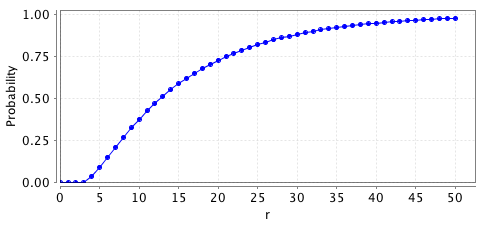### Example 3 (Molis)

In this example we consider the roommates instance from [KKW08] (Example 3, page 25), provided by Elena Molis. This instance concerns eight agents with the following preferences:

• a1 : a2,a3,a4,a6,a5,a7,a8
• a2 : a3,a1,a4,a5,a6,a8,a7
• a3 : a1,a2,a4,a5,a6,a7,a8
• a4 : a6,a3,a5,a1,a2,a7,a8
• a5 : a4,a7,a1,a2,a3,a6,a8
• a6 : a7,a4,a2,a3,a1,a5,a8
• a7 : a5,a6,a1,a2,a3,a4,a8
• a8 : a3

It is an unsolvable instance, it admits two stable half-matchings (with no even cycles), namely h1 and h2, where

• h1((4,5))=h1((6,7))=1 and h1((1,2))=h1((2,3))=h1((3,1))=1/2;

• h2((4,6))=h2((5,7))=1 and h2((1,2))=h2((2,3))=h2((3,1))=1/2.

The h1-stable matchings are:

• M1 = {(2,3) , (4,5) , (6,7)}
• M2 = {(1,2) , (4,5) , (6,7)}
• M3 = {(1,3) , (4,5) , (6,7)}

while the h2-stable matchings are:

• M4 = {(2,3) , (4,6) , (5,7)}
• M5 = {(1,2) , (4,6) , (5,7)}
• M6 = {(1,3) , (4,6) , (5,7)}

From results presented in [ILM08] it follows that, starting from any matching, we can always reach one of these matchings by successively satisfying blocking pairs. This implies that any ergodic set of the corresponding Markov chain must contain some of the above matchings.

The PRISM code for this instance is given below.

```// stable roommates example [KKW08]
// gxn 15/04/10

// we construct the model as a set of modules run in parallel, where each module corresponds to
// an agent and where the actions correspond to satisfying blocking pairs

// for example action eij corresponds to the current matching being updated due to agent i
// and j being a blocking pair, therefore the action should only be possible if both
// i and j satisfy the conditions of a blocking pair (this is indeed the case as the guards in
// the modules of agents i and j for action eij when combined match the condition of a blocking pair

// since satisfying a blocking pair can change the matching of other agents, we include these
// actions in the other agents so they can update there matching accordingly

// since PRISM automatically normalises the transition probabilities when constructing DTMCs,
// by giving each transition probability 1 (the default value so no probability needs to be
// verified), in the constructed model there will be a uniform probabilistic over the enabled transitions
// i.e. a uniform probabilistic choice between the current block pairs which corresponds precisely
// with the "better response dynamics" of Ackermann et al. [AGM+08] and the "unperturbed blocking dynamics"
// of Klaus et al. [KKW08]

// note we do not add loops in stable matchings, as using the fact that the stable matchings
// are deadlocks states (states with no outgoing transitions) we can use the label "deadlock"
// to specify the stable matchings, and hence simplify the specification of properties

dtmc // model is a DTMC/Markov chain

//------------------------------------------------------
// PREFERENCE LISTS
// agent i prefers j over k if aij>aik

const int a12=8;
const int a13=7;
const int a14=6;
const int a16=5;
const int a15=4;
const int a17=3;
const int a18=2;
const int a11=1;

const int a23=8;
const int a21=7;
const int a24=6;
const int a25=5;
const int a26=4;
const int a28=3;
const int a27=2;
const int a22=1;

const int a31=8;
const int a32=7;
const int a34=6;
const int a35=5;
const int a36=4;
const int a37=3;
const int a38=2;
const int a33=1;

const int a46=8;
const int a43=7;
const int a45=6;
const int a41=5;
const int a42=4;
const int a47=3;
const int a48=2;
const int a44=1;

const int a54=8;
const int a57=7;
const int a51=6;
const int a52=5;
const int a53=4;
const int a56=3;
const int a58=2;
const int a55=1;

const int a67=8;
const int a64=7;
const int a62=6;
const int a63=5;
const int a61=4;
const int a65=3;
const int a68=2;
const int a66=1;

const int a75=8;
const int a76=7;
const int a71=6;
const int a72=5;
const int a73=4;
const int a74=3;
const int a78=2;
const int a77=1;

const int a83=8;
const int a88=7;
const int a81=6;
const int a82=5;
const int a87=4;
const int a84=3;
const int a85=2;
const int a86=1;

//------------------------------------------------------
// constants used in renaming
const int N1=1;
const int N2=2;
const int N3=3;
const int N4=4;
const int N5=5;
const int N6=6;
const int N7=7;
const int N8=8;

//------------------------------------------------------
// module for first agent
module agent1

// current matching (start alone)
a1 : [1..8] init N1;

// wants to change matching
[e12] a1=0 | (a1=N1 & a12>a11)|(a1=N2 & a12>a12)|(a1=N3 & a12>a13)|(a1=N4 & a12>a14)|(a1=N5 & a12>a15)|(a1=N6 & a12>a16)|(a1=N7 & a12>a17)|(a1=N8 & a12>a18) -> (a1'=N2);
[e13] a1=0 | (a1=N1 & a13>a11)|(a1=N2 & a13>a12)|(a1=N3 & a13>a13)|(a1=N4 & a13>a14)|(a1=N5 & a13>a15)|(a1=N6 & a13>a16)|(a1=N7 & a13>a17)|(a1=N8 & a13>a18) -> (a1'=N3);
[e14] a1=0 | (a1=N1 & a14>a11)|(a1=N2 & a14>a12)|(a1=N3 & a14>a13)|(a1=N4 & a14>a14)|(a1=N5 & a14>a15)|(a1=N6 & a14>a16)|(a1=N7 & a14>a17)|(a1=N8 & a14>a18) -> (a1'=N4);
[e15] a1=0 | (a1=N1 & a15>a11)|(a1=N2 & a15>a12)|(a1=N3 & a15>a13)|(a1=N4 & a15>a14)|(a1=N5 & a15>a15)|(a1=N6 & a15>a16)|(a1=N7 & a15>a17)|(a1=N8 & a15>a18) -> (a1'=N5);
[e16] a1=0 | (a1=N1 & a16>a11)|(a1=N2 & a16>a12)|(a1=N3 & a16>a13)|(a1=N4 & a16>a14)|(a1=N5 & a16>a15)|(a1=N6 & a16>a16)|(a1=N7 & a16>a17)|(a1=N8 & a16>a18) -> (a1'=N6);
[e17] a1=0 | (a1=N1 & a17>a11)|(a1=N2 & a17>a12)|(a1=N3 & a17>a13)|(a1=N4 & a17>a14)|(a1=N5 & a17>a15)|(a1=N6 & a17>a16)|(a1=N7 & a17>a17)|(a1=N8 & a17>a18) -> (a1'=N7);
[e18] a1=0 | (a1=N1 & a18>a11)|(a1=N2 & a18>a12)|(a1=N3 & a18>a13)|(a1=N4 & a18>a14)|(a1=N5 & a18>a15)|(a1=N6 & a18>a16)|(a1=N7 & a18>a17)|(a1=N8 & a18>a18) -> (a1'=N8);

// one of the other pairs change so may need to update matching
[e23] true -> (a1'=(a1=N2 | a1=N3)?N1:a1);
[e24] true -> (a1'=(a1=N2 | a1=N4)?N1:a1);
[e25] true -> (a1'=(a1=N2 | a1=N5)?N1:a1);
[e26] true -> (a1'=(a1=N2 | a1=N6)?N1:a1);
[e27] true -> (a1'=(a1=N2 | a1=N7)?N1:a1);
[e28] true -> (a1'=(a1=N2 | a1=N8)?N1:a1);
[e34] true -> (a1'=(a1=N3 | a1=N4)?N1:a1);
[e35] true -> (a1'=(a1=N3 | a1=N5)?N1:a1);
[e36] true -> (a1'=(a1=N3 | a1=N6)?N1:a1);
[e37] true -> (a1'=(a1=N3 | a1=N7)?N1:a1);
[e38] true -> (a1'=(a1=N3 | a1=N8)?N1:a1);
[e45] true -> (a1'=(a1=N4 | a1=N5)?N1:a1);
[e46] true -> (a1'=(a1=N4 | a1=N6)?N1:a1);
[e47] true -> (a1'=(a1=N4 | a1=N7)?N1:a1);
[e48] true -> (a1'=(a1=N4 | a1=N8)?N1:a1);
[e56] true -> (a1'=(a1=N5 | a1=N6)?N1:a1);
[e57] true -> (a1'=(a1=N5 | a1=N7)?N1:a1);
[e58] true -> (a1'=(a1=N5 | a1=N8)?N1:a1);
[e67] true -> (a1'=(a1=N6 | a1=N7)?N1:a1);
[e68] true -> (a1'=(a1=N6 | a1=N8)?N1:a1);
[e78] true -> (a1'=(a1=N7 | a1=N8)?N1:a1);

endmodule

// construct further agents through renaming
module agent2=agent1[a1=a2, a11=a22, N1=N2, e12=e23, e13=e24, e14=e25, e15=e26, e16=e27, e17=e28, e18=e12, a12=a23, N2=N3, e23=e34, e24=e35, e25=e36, e26=e37, e27=e38, e28=e13, a13=a24, N3=N4, e34=e45, e35=e46, e36=e47, e37=e48, e38=e14, a14=a25, N4=N5, e45=e56, e46=e57, e47=e58, e48=e15, a15=a26, N5=N6, e56=e67, e57=e68, e58=e16, a16=a27, N6=N7, e67=e78, e68=e17, a17=a28, N7=N8, e78=e18, a18=a21, N8=N1 ] endmodule
module agent3=agent1[a1=a3, a11=a33, N1=N3, e12=e34, e13=e35, e14=e36, e15=e37, e16=e38, e17=e13, e18=e23, a12=a34, N2=N4, e23=e45, e24=e46, e25=e47, e26=e48, e27=e14, e28=e24, a13=a35, N3=N5, e34=e56, e35=e57, e36=e58, e37=e15, e38=e25, a14=a36, N4=N6, e45=e67, e46=e68, e47=e16, e48=e26, a15=a37, N5=N7, e56=e78, e57=e17, e58=e27, a16=a38, N6=N8, e67=e18, e68=e28, a17=a31, N7=N1, e78=e12, a18=a32, N8=N2 ] endmodule
module agent4=agent1[a1=a4, a11=a44, N1=N4, e12=e45, e13=e46, e14=e47, e15=e48, e16=e14, e17=e24, e18=e34, a12=a45, N2=N5, e23=e56, e24=e57, e25=e58, e26=e15, e27=e25, e28=e35, a13=a46, N3=N6, e34=e67, e35=e68, e36=e16, e37=e26, e38=e36, a14=a47, N4=N7, e45=e78, e46=e17, e47=e27, e48=e37, a15=a48, N5=N8, e56=e18, e57=e28, e58=e38, a16=a41, N6=N1, e67=e12, e68=e13, a17=a42, N7=N2, e78=e23, a18=a43, N8=N3 ] endmodule
module agent5=agent1[a1=a5, a11=a55, N1=N5, e12=e56, e13=e57, e14=e58, e15=e15, e16=e25, e17=e35, e18=e45, a12=a56, N2=N6, e23=e67, e24=e68, e25=e16, e26=e26, e27=e36, e28=e46, a13=a57, N3=N7, e34=e78, e35=e17, e36=e27, e37=e37, e38=e47, a14=a58, N4=N8, e45=e18, e46=e28, e47=e38, e48=e48, a15=a51, N5=N1, e56=e12, e57=e13, e58=e14, a16=a52, N6=N2, e67=e23, e68=e24, a17=a53, N7=N3, e78=e34, a18=a54, N8=N4 ] endmodule
module agent6=agent1[a1=a6, a11=a66, N1=N6, e12=e67, e13=e68, e14=e16, e15=e26, e16=e36, e17=e46, e18=e56, a12=a67, N2=N7, e23=e78, e24=e17, e25=e27, e26=e37, e27=e47, e28=e57, a13=a68, N3=N8, e34=e18, e35=e28, e36=e38, e37=e48, e38=e58, a14=a61, N4=N1, e45=e12, e46=e13, e47=e14, e48=e15, a15=a62, N5=N2, e56=e23, e57=e24, e58=e25, a16=a63, N6=N3, e67=e34, e68=e35, a17=a64, N7=N4, e78=e45, a18=a65, N8=N5 ] endmodule
module agent7=agent1[a1=a7, a11=a77, N1=N7, e12=e78, e13=e17, e14=e27, e15=e37, e16=e47, e17=e57, e18=e67, a12=a78, N2=N8, e23=e18, e24=e28, e25=e38, e26=e48, e27=e58, e28=e68, a13=a71, N3=N1, e34=e12, e35=e13, e36=e14, e37=e15, e38=e16, a14=a72, N4=N2, e45=e23, e46=e24, e47=e25, e48=e26, a15=a73, N5=N3, e56=e34, e57=e35, e58=e36, a16=a74, N6=N4, e67=e45, e68=e46, a17=a75, N7=N5, e78=e56, a18=a76, N8=N6 ] endmodule
module agent8=agent1[a1=a8, a11=a88, N1=N8, e12=e18, e13=e28, e14=e38, e15=e48, e16=e58, e17=e68, e18=e78, a12=a81, N2=N1, e23=e12, e24=e13, e25=e14, e26=e15, e27=e16, e28=e17, a13=a82, N3=N2, e34=e23, e35=e24, e36=e25, e37=e26, e38=e27, a14=a83, N4=N3, e45=e34, e46=e35, e47=e36, e48=e37, a15=a84, N5=N4, e56=e45, e57=e46, e58=e47, a16=a85, N6=N5, e67=e56, e68=e57, a17=a86, N7=N6, e78=e67, a18=a87, N8=N7 ] endmodule

//------------------------------------------------------
// reward structure: expected rounds
rewards "rounds"
true : 1;
endrewards
```

Constructing this instance in PRISM, we find there are 308 matchings and a single ergodic set:

• 43:(1,3,2,6,7,4,5,8)
• 109:(2,1,3,6,7,4,5,8)
• 135:(2,1,8,6,7,4,5,3)
• 145:(3,2,1,6,7,4,5,8)

which corresponds to the matchings M4, M5, M7(={(1,2) , (3,8) , (4,6) , (5,7)}) and M6 respectively. This corresponds to the results presented in [KKW08]. Computing the long-term likelihood of being in any one of the matching (i.e. the steady state probabilities of the Markov chain) we find:

• 43:(1,3,2,6,7,4,5,8)=0.285714
• 109:(2,1,3,6,7,4,5,8)=0.285714
• 135:(2,1,8,6,7,4,5,3)=0.142857
• 145:(3,2,1,6,7,4,5,8)=0.285714

We now study the converge of the instance through the following properties:

• the probability of reaching the ergodic set within r rounds;

• the expected number of rounds to reach the ergodic set.

For this roommates instance, these properties can be expressed in PRISM as follows.

```// prism find a single ergodic set (BSCC) specified by the following atomic proposition
label "ergodic1" = (a1=1 & a2=3 & a3=2 & a4=6 & a5=7 & a6=4 & a7=5 & a8=8) |
(a1=2 & a2=1 & a3=3 & a4=6 & a5=7 & a6=4 & a7=5 & a8=8) |
(a1=2 & a2=1 & a3=8 & a4=6 & a5=7 & a6=4 & a7=5 & a8=3) |
(a1=3 & a2=2 & a3=1 & a4=6 & a5=7 & a6=4 & a7=5 & a8=8);

// probability reached a ergodic set by round R
const int r; // bound of number of rounds
P=?[ F<=r "ergodic1" ]

// expected number of rounds to reach the ergodic set
R{"rounds"}=? [ F "ergodic1" ]
```

Analysing these properties in PRISM we find the expected number of rounds equals 16.49788 and the probabilities are plotted in the graph below.### Case study

We now compare the performance characteristics of a number of different instances of the SM problem, as the number of men and women k(=n/2) varies between 4 and 8.

• Symmetric: in this instance the preferences of the men and women are of the form:

• mj : wj,...,wk,w1,...,w(j-1)
• wj : mj,...,mk,m1,...,m(j-1).
• Uncoord: this instance is used in [AGM+08] to show an exponential lower bound for the convergence time. The preference lists in this instance are given by:

• mj : w(j+1),...,wk,w1,...,wj
• wj : mj,m(j+1),...,mk,m1,..,m(j-1).
• Uniform: in this case the preference lists of all men and all women are the same and equal w1,w2,...,wk and m1,m2,...,m_k respectively.

For comparison, in our results we also includes the minimum, average and maximum values obtained from a sample of 1,000 random instances for k equal to 4, 5, 6 and 7.

The specification for these instances follow those for Examples 1 and 2. To simplify this construction, we use the PRISM preprocessing language. For example, the specification in the PRISM preprocessing language for the Uncoord instances is given below.

```// stable marriage uncoord instance
// gxn 17/04/10

#const N#

dtmc

//------------------------------------------------------
// PREFERENCE LISTS
// man i prefers woman j over woman k if mij>mik
// woman i prefers man j over man k if wij>wik

// preference list for men
#for i=1:N#
#for j=1:N#
const int m#i##j#=#N-mod((N-1)-((j-1)-i),N)#;
#end#

#end#

// preference list for women
#for i=1:N#
#for j=1:N#
const int w#i##j#=#mod((N-1)-((i-1)-j),N)+1#;
#end#

#end#

//------------------------------------------------------
// constants used in renaming
#for i=1:N#
const int N#i#=#i#;
#end#

//------------------------------------------------------
// module for first man
module man1

// current preferences (0 means no preference)
m1 : [0..#N#];

// man 1 wants to change
#for i=1:N#
[e1#i#] m1=0 | #| j=1:N#(m1=#j# & m1#i#>m1#j#)#end# -> (m1'=#i#);
#end#

// one of the other pairs change so may need to reset its choice
#for i=1:N#
#for j=2:N#
[e#j##i#] true -> (m1'=(m1=#i#)?0:m1);
#end#
#end#

endmodule

// construct further men through renaming
#for i=2:N#
module man#i#=man1[m1=m#i#, #, j=1:N# m1#j#=m#i##j#, #, k=1:N# e#j##k#=e#mod(j-1+i-1,N)+1##k# #end##end# ] endmodule
#end#

//------------------------------------------------------
// module for first woman
module woman1

// do not need a preference (can work it out from the men's preference)

// man 1 wants to change
#for i=1:N#
[e#i#1] (#& j=1:N# m#j#!=N1 #end#) | #| j=1:N#(m#j#=N1 & w1#i#>w1#j#)#end# -> true;
#end#

endmodule

// construct further women through renaming
#for i=2:N#
module woman#i#=woman1[ N1=N#i#, #, j=1:N# w1#j#=w#i##j#, #, k=1:N# e#k##j#=e#k##mod(j-1+i-1,N)+1# #end##end# ] endmodule
#end#

//------------------------------------------------------
// initial states: all complete matching
init
#& i=1:N##& j=i+1:N# m#i#!=m#j# #end# #end# // each man is matched to a diiferent woman
#& i=1:N# m#i#>0 #end# // all men are matched
endinit
//------------------------------------------------------
// reward structure: expected steps
rewards "rounds"
true : 1;
endrewards
```

In the specification above we suppose that the system starts with a (random) complete matching. In our experiments we also consider the case when the system starts in the empty matching. This case can be specified by simply removing the initial state specification and then (by default) in the initial state each variable takes its least value, i.e. each variable is initialised with the value 0.

##### Model Statistics

The table below shows the model statistics for the different instances we have built when starting from a random complete matching.

• Specific Instances:

 N: Symmetric: Uncoord: Uniform: states: transitions: states: transitions: states: transitions: 4 208 1,433 208 1,268 87 369 5 1,545 15,901 1,545 14,205 665 4,746 6 13,326 189,691 13,326 170,886 5,972 64,341 7 130,921 2,450,001 130,921 2,222,745 61,215 926,095 8 1,441,728 34,194,273 1,441,728 31,209,032 702,311 14,175,310
• 1,000 random samples:

 N: Minimum: Average: Maximum: states: transitions: states: transitions: states: transitions: 4 102 461 193 1,247 208 1,460 5 993 8,524 1,562 15,618 1,545 16,660 6 9,272 119,035 13,317 192,465 13,326 202,560 7 130,884 2,378,889 130,918 2,524,157 130,921 2,657,024

When starting from the empty matching, all instances gave the same model statistics which are given in the following table.

 N: states: transitions: 4 1,284 28.04 5 14,230 97.16 6 170,922 416.5 7 2,222,794 2,220 8 31,209,096 14,388

##### Stable Matchings

We first consider the number of different stable matchings of instances, which correspond to the number of deadlock states in each PRISM model. In the tables below we give these results for each the different instances and also the minimum, average and maximum number of stable matchings for the 1,000 randomly generated instances of each size.

• Specific Instances:

 N: Stable Matchings: Symmetric: Uncoord: Uniform: 4 1 4 1 5 1 5 1 6 1 6 1 7 1 7 1 8 1 8 1
• 1,000 random samples:

 N: Stable Matchings: Minimum: Average: Maximum: 4 1 1.506 5 5 1 1.657 5 6 1 1.961 7 7 1 2.187 9

##### Convergence

To study the convergence of the different instances we consider:

• the probability of reaching a stable matching within r rounds when starting from the empty matching;

• the expected number of rounds to reach a stable matching both when starting from a random complete matching and starting from an empty matching.

These properties can be expressed in PRISM as follows.

```// probability of reaching a stable matching within r rounds
const int r;

// expected rounds to reach a stable matching
filter(max,R=?[ F "deadlock" ],"init") // maximum value over initial states
filter(avg,R=?[ F "deadlock" ],"init") // average value over initial states
```

Notice that to compute the average and maximum value of the expected rounds we use the filter command which is currently only available in a prototype version of PRISM (however it will be included in the next release of PRISM).

Concerning the probability of reaching a stable matching within r rounds, the following graphs plot these results for each of the specific instances.

• Symmetric: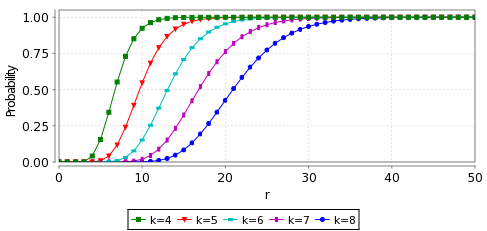• Uncoord:• Uniform: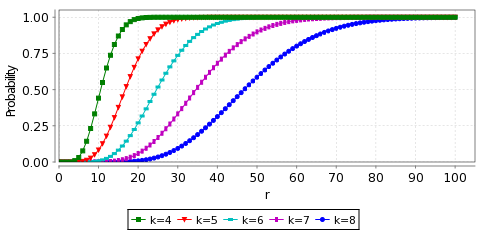With regards to the expected time properties, the tables below give the expected number of rounds to reach a stable matching for each of the specific instances and also the minimum, average and maximum values over 1,000 random samples. We have also included plots showing how the values differ over the random samples for the expected number of rounds when starting from the empty initial matching.

• Specific Instances:

 N: expected rounds to reach a stable matching: complete initial matching: empty initial matching: Symmetric: Uncoord: Uniform: Symmetric: Uncoord: Uniform: av.: max: av.: max: av.: max: 4 3.595 5.713 18.97 25.22 6.822 9.160 7.469 28.04 11.31 5 5.456 7.919 84.23 93.74 12.04 14.92 10.51 97.16 17.66 6 7.692 11.05 399.2 413.5 19.08 22.73 13.95 416.5 25.82 7 10.30 14.09 2,197 2,216 28.18 32.42 17.78 2,220 36.03 8 13.27 17.97 14,361 14,385 39.61 44.56 21.99 14,388 48.56
• Minimum of 1,000 random samples:

 N: expected rounds to reach a stable matching: complete initial matching: empty initial matching: av.: max: 4 4.735 6.932 7.851 5 8.082 11.21 11.53 6 11.61 15.59 15.77 7 15.93 20.89 21.04
• Average of 1,000 random samples:

 N: expected rounds to reach a stable matching: complete initial matching: empty initial matching: av.: max: 4 8.032 10.65 11.02 5 13.83 17.34 17.54 6 22.84 27.28 27.39 7 37.34 42.74 42.80
• Maximum of 1,000 random samples:

 N: expected rounds to reach a stable matching: complete initial matching: empty initial matching: av.: max: 4 17.25 20.30 20.35 5 46.28 50.68 50.61 6 115.8 121.1 121.2 7 164.9 170.7 170.8
• Plots of the expected number rounds when starting from the empty initial matching for the 1,000 random samples.

• k=4• k=5• k=6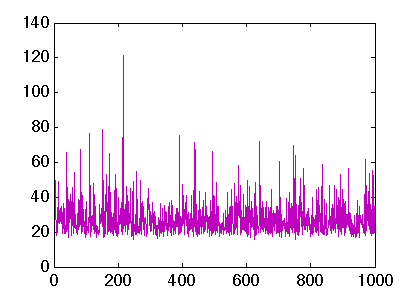• k=7